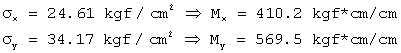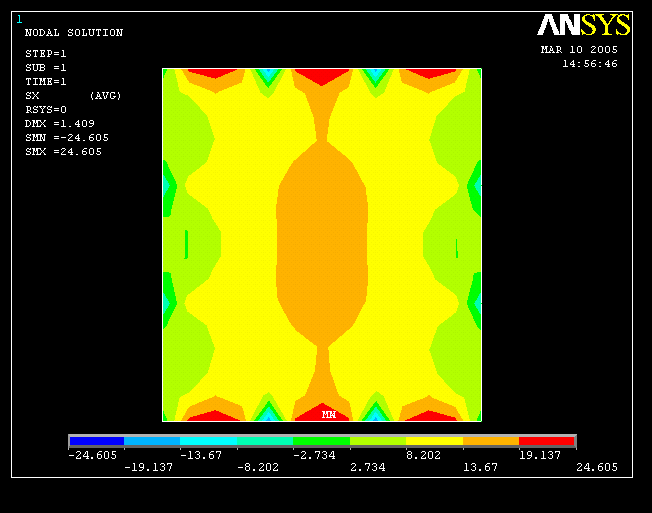# Anechoic chamber

The chamber is supported by springs on its perimeter. The design live load for the floor is equal to 250 kgf/m2. The design live load for the ceiling is equal to 50 kgf/m2.

Specific weight (concrete) = 2320.0 kgf/m3
Surface (ceiling) = 8.1 m2
Volume (wall, ceiling) = 4.0 m3
Wall perimeter = 11.80 m

## Spring

FDS-1-5730-B
rated capacity = 2599 kgf
rated deflection = 2.5 cm
k = 1.0396E+03

# Model 1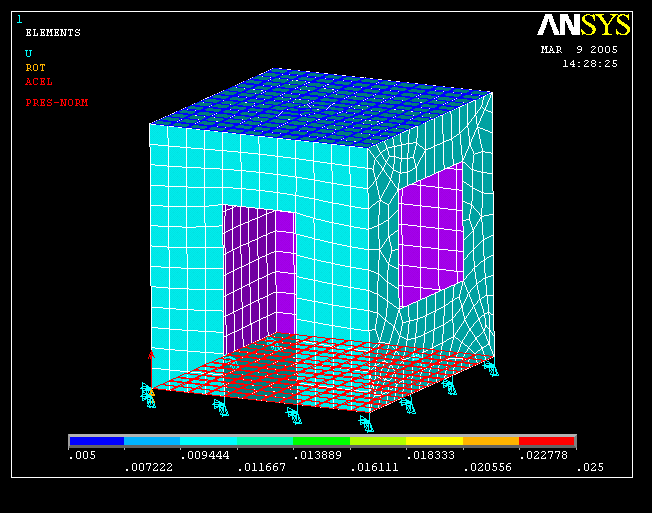## Displacements

The following table shows the vertical displacements of the top corner nodes, counterclockwise starting at the node on the left side of the door.

 Node Displ Z 251 -1.1 cm 230 -1.0 cm 418 -1.1 cm 536 -1.2 cm

## Reactions

The following table shows the vertical reactions of the bottom nodes, counterclockwise starting at the node on the left side of the door.

 Node Force Z 1032 1179.0 kgf 1033 1137.9 kgf 1034 1096.8 kgf 1035 1056.2 kgf 1036 1094.9 kgf 1037 1133.7 kgf 1038 1171.9 kgf 1039 1213.3 kgf 1040 1254.5 kgf 1041 1294.7 kgf 1042 1256.9 kgf 1043 1218.1 kgf

## Stresses (floor)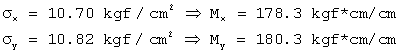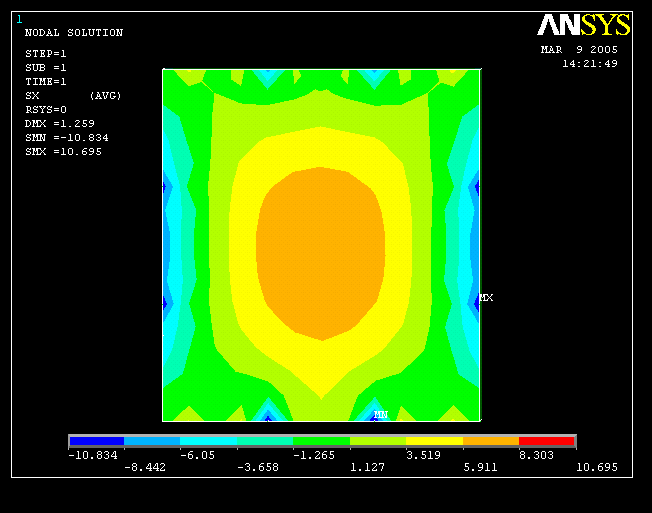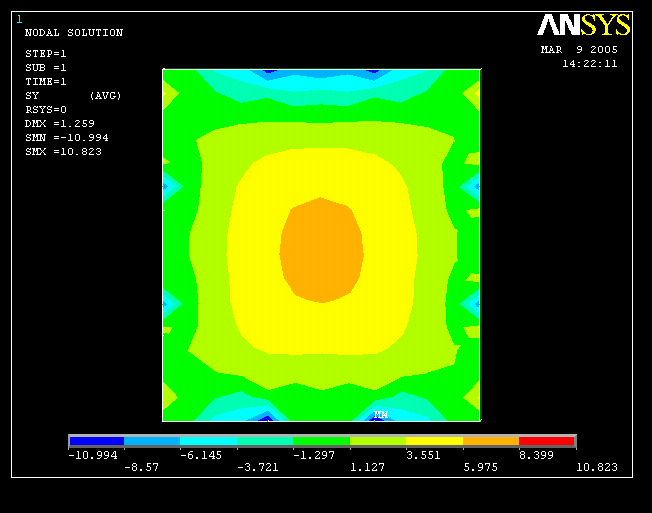## Vibration frequencies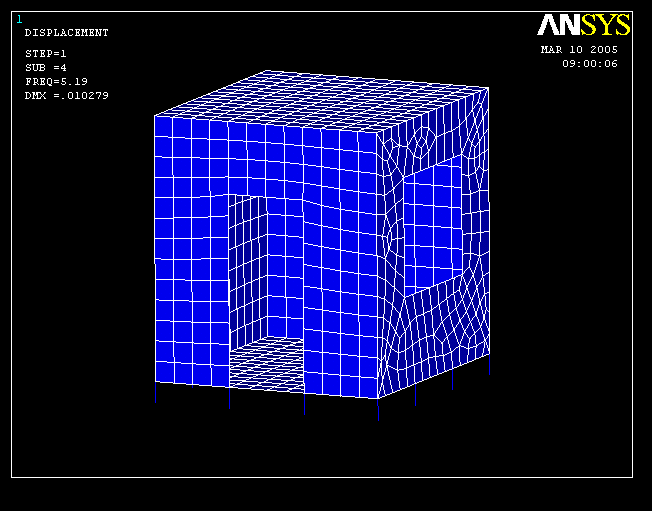# Model 2

The weight of the wall, the weight and the live load of the ceiling were applied as force on the edges of the floor. The force/length on the edge can be calculated as (4.0 * 2320.0 + 8.1 * 50) / 11.80 = 821 kgf/m.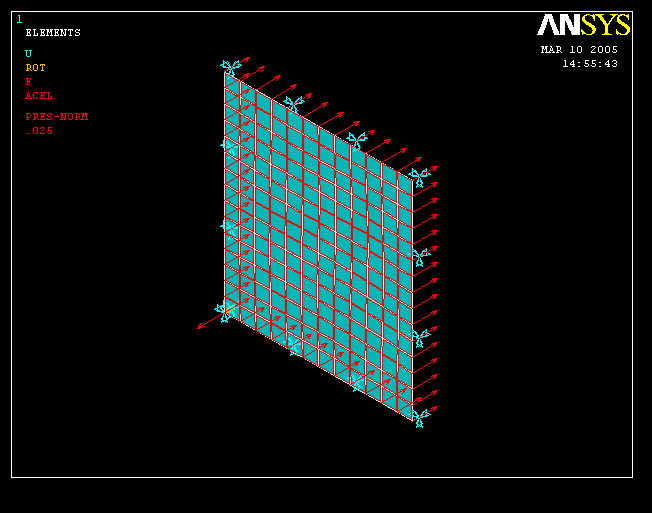## Stresses (floor)